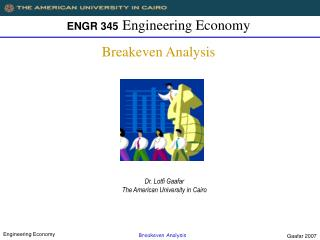DownloadDownload PresentationENGR 345 Engineering Economy Breakeven Analysis

# ENGR 345 Engineering Economy Breakeven Analysis

Download Presentation## ENGR 345 Engineering Economy Breakeven Analysis

- - - - - - - - - - - - - - - - - - - - - - - - - - - E N D - - - - - - - - - - - - - - - - - - - - - - - - - - -
##### Presentation Transcript

1. ENGR 345Engineering EconomyBreakeven Analysis Dr. Lotfi GaafarThe American University in Cairo Gaafar 2007

2. Introduction to Breakeven Analysis • Breakeven Analysis in the context of Production planning addresses the decision of whether to make or buy a product. • Making the product involves two cost elements: • Fixed costs such as machine renting cost and operation expenses • Variable costs such as raw material cost • Buying the product involves only one cost element, the selling price. However, the price may either be constant or variable based on the quantity. Gaafar 2007

3. Linear Breakeven Analysis Assumptions: (1) Price is constant (p); (2) We have fixed costs; (3) We have constant variable cost. Buy Cost (BC) = pQ Making is better Cost Make Cost (MC) = FC + vQ Buying is better Variable cost: vQ Fixed Cost: FC Savings : S = BC – MC = pQ – FC – vQ Breakeven: S = 0, pQ = FC + vQ Quantity (Q) Q’ Gaafar 2007

4. Linear Breakeven Analysis A particular product sells for a price of \$70. If the fixed cost are \$800/month and the variable cost per unit is \$30/unit, determine the breakeven quantity.  Gaafar 2007

5. Nonlinear Breakeven Analysis Assumptions: (1) Price varies with demand; (2) We have fixed costs; (3) We have constant variable cost. \$ Make Cost (MC) = FC + vQ Buy Cost (BC) = pQ Break-even output maximum savings Break-even output Fixed Cost: FC p = (a – bQ) • Savings (S) = pQ – FC – vQ = (a – bQ)Q – FC – vQ • S = aQ – bQ2 – (FC+vQ) • S = (a-v)Q – bQ2 –FC ………….. (1) • Set (1) equal to zero to determine breakeven. • Differentiate (1) with respect to Q and set the result equal to zero to find Q that maximizes the savings. Gaafar 2007

6. Nonlinear Breakeven Analysis A particular product has a unit price that starts at \$78 with a \$0.1 discount for each unit purchased. If the fixed cost are \$800/month and the variable cost per unit is \$30/unit, determine the demand quantity that maximizes the savings of making and the breakeven quantities. [240, 17.28, 462.72] Gaafar 2007

7. Time Value of Money Suppose a firm is considering manufacturing a new product and the following data have been provided: Sales price \$12.50/unit Equipment cost \$200,000 Overhead cost \$50,000/year Operating and maintenance cost \$25/operating hour Production time/1000 units 100 hours Planning horizon 5 years Minimum attractive rate of return 15% Determine the sales volume that would make manufacturing this product profitable. [10,966] Gaafar 2007

8. Time Value of Money Consider a contractor who experiences a seasonal pattern of activity for compressors. The manager currently owns eight compressors and suspects that this number will not be adequate to meet the demand. The contractor realizes that there will arise situations when more than eight compressors will be required, and is considering purchasing an additional compressor for use during heavy demand periods. Local equipment rental firm will rent compressors at a cost of \$50/day. Compressors can be purchased for \$6000. The difference in operating and maintenance costs between owned and rented compressors is estimated to be \$3000/year. Letting X denote the number of days a year that more than eight compressors are required, perform a breakeven analysis. Assume a planning horizon of 5 years, zero salvage values, and 20% minimum attractive rate of return. [100.128] Gaafar 2007

9. Basic Definitions • Fixed/Variable Costs - If costs change appreciably with fluctuations in business activity, they are “variable.” Otherwise, they are “fixed.” A widely used cost model is: Total Costs = Fixed Costs + Variable Costs • Price is the amount of money, goods or services that must be given up to acquire ownership or use of a product. • Recurring/Nonrecurring Costs are repetitive and occur when an organization produces goods or services on a continuing basis, they are “recurring.” Otherwise they are “nonrecurring.” Variable costs are recurring since they repeat with each unit of output. • Direct/Indirect Costs - If costs can be reasonably measured and allocated to a specific output, they are “direct.” Otherwise they are “indirect.” Gaafar 2007

10. Basic Definitions • Overhead Costs - All costs of providing goods or services other than direct labor and direct material. Indirect costs are a subset of overhead costs. Fixed overhead relates more to plant capacity than production volume (variable overhead). Allocation of overhead to specific outputs may be in proportion to: 1. Direct labor hours 2. Direct material costs 3. Machine hours • Opportunity Costs - The cost of forgoing the chance to earn interest (or profit) on investment funds. Gaafar 2007